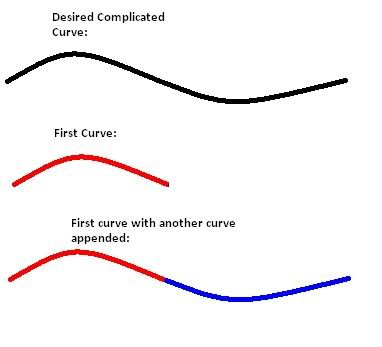# DiscussionB-Splines and Bezier Curves

#### Sevion

##### The DIY Ninja
Hello, people. I have a problem.

I've never studied B-Splines or Bezier Curves.

I got this so far:

JASS:
``````	private function Bezier takes real r, real a1, real c1, real a2, real c2 returns real
return r * r * r * ( a1 + 3 * ( c1 - c2 ) - a1 ) + 3 * r * r * a1 - 2 * c1 + c2 ) + 3 * r * ( c1 - a1 ) + a1
endfunction``````

That only works for two lines though. How can I get more than two lines?

I saw somewhere about multiplying (r + 1), but I haven't tried it.

Bump.

#### emjlr3

##### Change can be a good thing
Staff member
could you elaborate just a bit? what exactly are your trying to accomplish?

the way I always used bezier curves for horizontal parabolic movements was as follows:
JASS:
``````       local real Ax=GetUnitX(caster)
local real Ay=GetUnitY(caster)
local real Cx=GetSpellTargetX()
local real Cy=GetSpellTargetY()
// a positive angle would create a mirrored curve along with y axis(path toward target)
local real angle = -45.
// horizontal width would be how big(steep, larger slope) of a parabola you wanted
local real Bx = Ax+horizontalwidth*Cos(Atan2(Cy-Ay,Cx-Ax)-angle)
local real By = Ay+horizontalwidth*Sin(Atan2(Cy-Ay,Cx-Ax)-angle)
local real a = 1.

// update desired x/y coordinates along curve path periodically
local real b=1.-a
local real x = Ax*a*a+Bx*2.*a*b+Cx*b*b
local real y = Ay*a*a+By*2.*a*b+Cy*b*b

// update missile loc periodically
call SetUnitX(missile,x)
call SetUnitY(missile,y)
// decrease a periodically as desired to move the missile along the path of the curve
set a=a-.02``````

hope that helps some, might not be what you want or need though, not really sure what you need when saying more then two curves...

#### Sevion

##### The DIY Ninja
Well, what I mean by more than two lines is that my current function only allows for a single pair of lines (Quadratic).

How can I apply this to allow for more than two anchor and control coordinate sets?

I.E. the bottom right curve in this image:#### uberfoop

Is there an issue with simply splining the curves together? One of the main reasons to use curves with easily forced ending directions is that you can very easily build a spline with continuous first derivative (I specifically say "first derivative", because things like second derivative and curvature hate people, as I discovered when building my camera systems; even if a bezier splint joint looks smooth, and even if you camera can be moved along the spline joint smoothly, things can go quite awry if you try to use the curve tangent as your camera eye facing direction as you move the camera along the curve).

#### Sevion

##### The DIY Ninja
So it's possible to just use the same function using the second line as the first line for the second curve?

I wasn't sure, but I thought that would lead to some overlap. But now that I think about it, ofc you would just use the midpoint of the first line, right?

#### uberfoop

So it's possible to just use the same function using the second line as the first line for the second curve?

I wasn't sure, but I thought that would lead to some overlap. But now that I think about it, ofc you would just use the midpoint of the first line, right?
I'm not entirely sure what you mean, but basically, it works kind of like this:•1 person

#### Sevion

##### The DIY Ninja
Yeah. I wasn't thinking all the way through. But now I understand ^_^ Thanks for clearing this up.

#### emjlr3

##### Change can be a good thing
Staff member
personally - I would simply research a universal function for the curve fit I desired, and extrapolate that into wc3 script

ie, a simple quadratic with form ax2 + bx + c could easily be adjusted to allow for parabolic movement about the y-axis, where x is distance to target, y would be the scalar distance from the y axis (or the x value, if looking at a graph), and the two points, x/y, would give you your coordinates for that point along your curve

the same could be done for any curve shape, so long as you have a function that predicts such movement accurately

any who, that would be my approach, rather then attempting to splice together unrelated functions

General chit-chat
Help Users
• No one is chatting at the moment.

### Members online

No members online now.